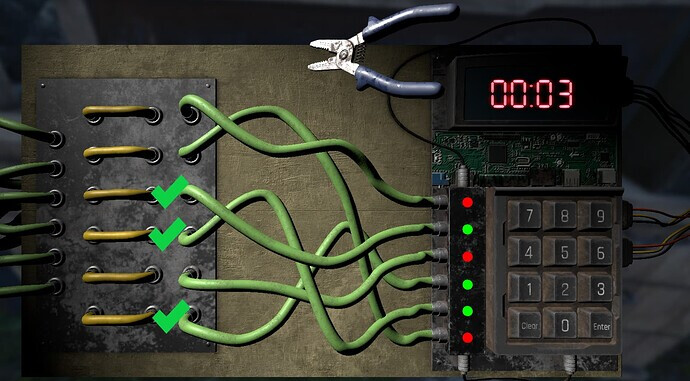# Tracing path of wires

``````from skimage.morphology import skeletonize
from skimage import data
from skimage import io
import skimage
import matplotlib.pyplot as plt
from skimage.util import invert

# Invert the horse image

# perform skeletonization
skeleton = skeletonize(image)

# display results
fig, axes = plt.subplots(nrows=1, ncols=2, figsize=(8, 4),
sharex=True, sharey=True)

ax = axes.ravel()

ax.imshow(image, cmap=plt.cm.gray)
ax.axis('off')
ax.set_title('original', fontsize=20)

ax.imshow(skeleton, cmap=plt.cm.gray)
ax.axis('off')
ax.set_title('skeleton', fontsize=20)

fig.tight_layout()
plt.show()
``````I am trying to trace the path of the wires like in the image above, that way I can automate a bomb-defusing mini-game. In order to win the mini-game, the player has to follow the wires with a green light next to the to the other end and cut the wire. I am trying to start by isolating the wire paths and thought using scikit-image skeletonize would be a good start.

I tried skeletonizing the above image and some other images that isolate the wires and have less distracting objects, but only the one with the check-marks at the end of the wires resulted in an output.
But this image took a while to process, so I wanted to try isolating just the wires to reduce processing times. Hence the smaller image with just wires, but when doing so, both result in an empty output like this:

Does anyone know how to solve the issue where I dont get any output, and would anyone know a more efficient way of figuring out the paths of the wire?